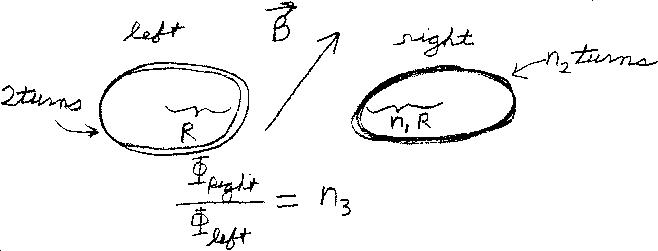Problem C7: The two circular loops of wire lie in the same plane. The loop on the left has two turns of wire and a radius R. The loop on the right has n2 turns of wire and a radius of n1R. There is a constant magnetic field in the whole region that does not lie in the plane of the wire loops. What is the ratio of the magnetic flux through the right wire divided by the magnetic flux through the left wire? If Φright / Φleft = n3, what is n3?n1 = n2 = Input n3:
If you are currently in my class, you can record your grade by entering your name and student ID number (without the leading zeros) below and clicking on "record grade".
 First Name = Last Name = ID = Problem: